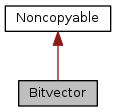ARB
Bitvector Class Reference

`#include <MultiProbe.hxx>`

Inheritance diagram for Bitvector:[legend]
Collaboration diagram for Bitvector:[legend]

## Public Member Functions

int gen_id ()

Bitvectormerge (Bitvector *x)

int subset (Bitvector *Obermenge)

int setbit (int pos)

int delbit (int pos)

void rshift ()

void print ()

charget_vector ()

int get_num_of_bits ()

int get_len ()

void set_vector (char *back)

Bitvector (int bits)

~Bitvector ()

## Detailed Description

Definition at line 338 of file MultiProbe.hxx.

## Constructor & Destructor Documentation

 Bitvector::Bitvector ( int bits )

Definition at line 15 of file MP_permute.cxx.

Referenced by merge().

 Bitvector::~Bitvector ( )

Definition at line 26 of file MP_permute.cxx.

## Member Function Documentation

 int Bitvector::gen_id ( )

Definition at line 31 of file MP_permute.cxx.

 Bitvector * Bitvector::merge ( Bitvector * x )

Definition at line 38 of file MP_permute.cxx.

References Bitvector(), get_num_of_bits(), readbit(), and setbit().

 int Bitvector::subset ( Bitvector * Obermenge )

Definition at line 54 of file MP_permute.cxx.

References get_vector().

 int Bitvector::readbit ( int pos )

Definition at line 111 of file MP_permute.cxx.

Referenced by delbit(), gen_id(), merge(), print(), and rshift().

 int Bitvector::setbit ( int pos )

Definition at line 88 of file MP_permute.cxx.

Referenced by merge(), and rshift().

 int Bitvector::delbit ( int pos )

Definition at line 99 of file MP_permute.cxx.

 void Bitvector::rshift ( )

Definition at line 66 of file MP_permute.cxx.

 void Bitvector::print ( )

Definition at line 80 of file MP_permute.cxx.

Referenced by Sonde::print().

 char* Bitvector::get_vector ( )
inline

Definition at line 353 of file MultiProbe.hxx.

Referenced by subset().

 int Bitvector::get_num_of_bits ( )
inline

Definition at line 354 of file MultiProbe.hxx.

Referenced by merge().

 int Bitvector::get_len ( )
inline

Definition at line 355 of file MultiProbe.hxx.

 void Bitvector::set_vector ( char * back )
inline

Definition at line 357 of file MultiProbe.hxx.

The documentation for this class was generated from the following files: